## 排序相描述

1. 排序分类：若排序过程中，所有的文件都是放在内存中处理的，不涉及数据的内外存交换，则称该排序算法是内部排序算法; 若排序过程中涉及内外存交换，则是外部排序。内部排序适合小文间，外部排序适用于不能一次性把所有记录放入内存的大文件。常见的分类算法还可以根据排序方式分为两大类：比较排序非比较排序
2. 排序算法的稳定性： 若排序对象中存在多个关键字相同的记录，经过排序后，相同关键字的记录之间的相对次序保持不变，则该排序方法是稳定的，若次序发生变化(哪怕只有两条记录之间)，则该排序方法是不稳定的。关于哪些算法是稳定的，哪些不稳定，下面会详细介绍。
3. 时间复杂度：一般情况下，算法中基本操作重复执行的次数是问题规模$n$的某个函数，用$T(n)$表示，若有某个辅助函数$f(n)$，使得$T(n)/f(n)$的极限值（当$n$趋近于无穷大时）为不等于零的常数，则称$f(n)$是$T(n)$的同数量级函数。记作$T(n)=O(f(n))$，称$O(f(n)) ​$为算法的渐进时间复杂度，简称时间复杂度。
4. 空间复杂度：空间复杂度(Space Complexity)是对一个算法在运行过程中临时占用存储空间大小的量度，它是问题规模$n$的函数，记做$S(n)=O(f(n))$。比如直接插入排序的时间复杂度是$O(n^2)$,空间复杂度是$O(1)$。而一般的递归算法就要有$O(n)$的空间复杂度了，因为每次递归都要存储返回信息，需要辅助空间的大小随着$n$的增大线性增大。
5. 就地排序：若一个排序算法所需的辅助空间并不依赖于问题的规模n，即时间复杂度是$O(1)$,则称该排序算法为就地排序。非就地排序算法的时间复杂度一般为$O(n)$。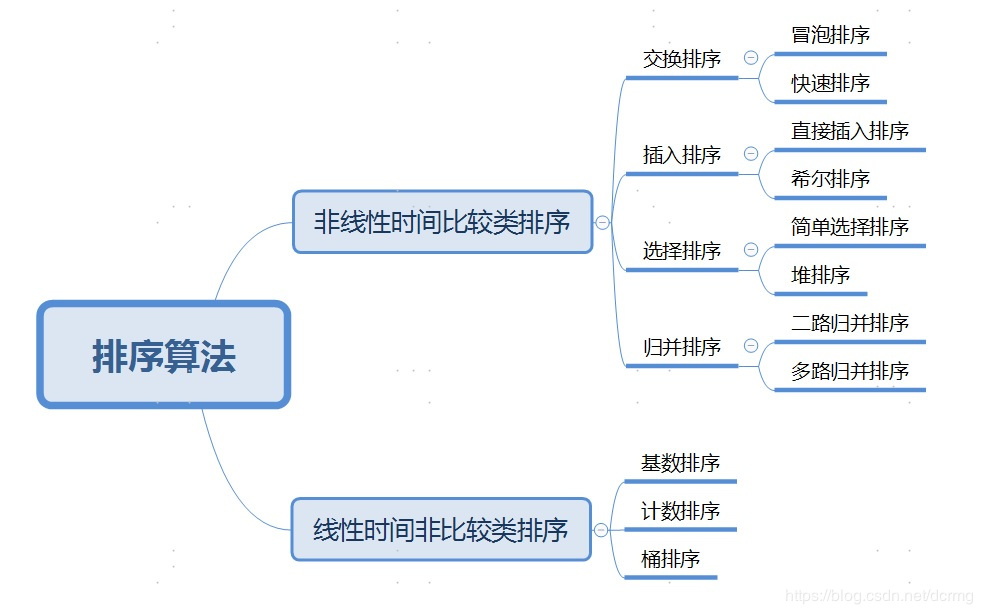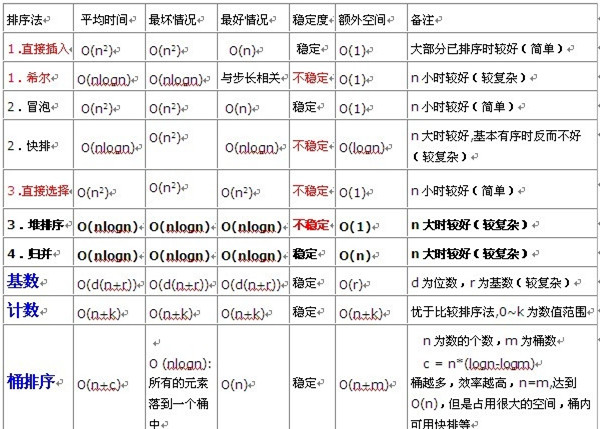## 直接插入排序（Insertion Sort）

### 算法流程

1. 初始时， $a$自成一个有序区， 无序区为$a[1, … , n-1]$, 令$i=1$;
2. 将$a[i]$并入当前的有序区$a[0, … , i-1]$;
3. $i++$并重复步骤2，直到$i=n-1$, 排序完成。

1. 从第一个元素开始，该元素可以认为已经被排序
2. 取出下一个元素，在已经排序的元素序列中从后向前扫描
3. 如果该元素（已排序）大于新元素，将该元素移到下一位置
4. 重复步骤3，直到找到已排序的元素小于或者等于新元素的位置
5. 将新元素插入到该位置中
6. 重复步骤2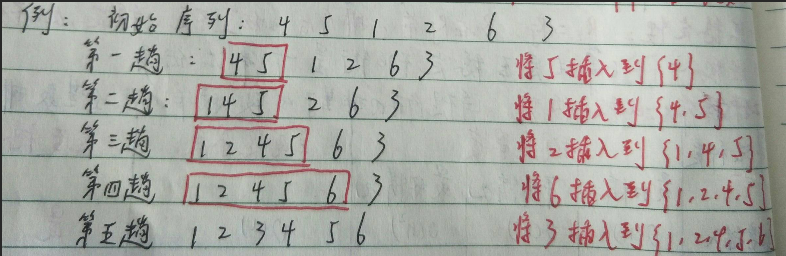## 希尔排序（Shell Sort）

### 基本思想

1. 希尔排序是把记录按下标的一定量分组，对每组使用直接插入算法排序；
2. 随着增量逐渐减少，每组包1含的关键词越来越多，当增量减至1时，整个文件恰被分成一组，算法被终止。

### 算法流程

1. 选择一个增量序列$t_1，t_2，…，t_k$，其中$t_i>t_j，t_k=1$；
2. 按增量序列个数$k$，对序列进行$k$趟排序；
3. 每趟排序，根据对应的增量$t_i$，将待排序列分割成若干长度为$m$ 的子序列，分别对各子表进行直接插入排序。仅增量因子为1 时，整个序列作为一个表来处理，表长度即为整个序列的长度。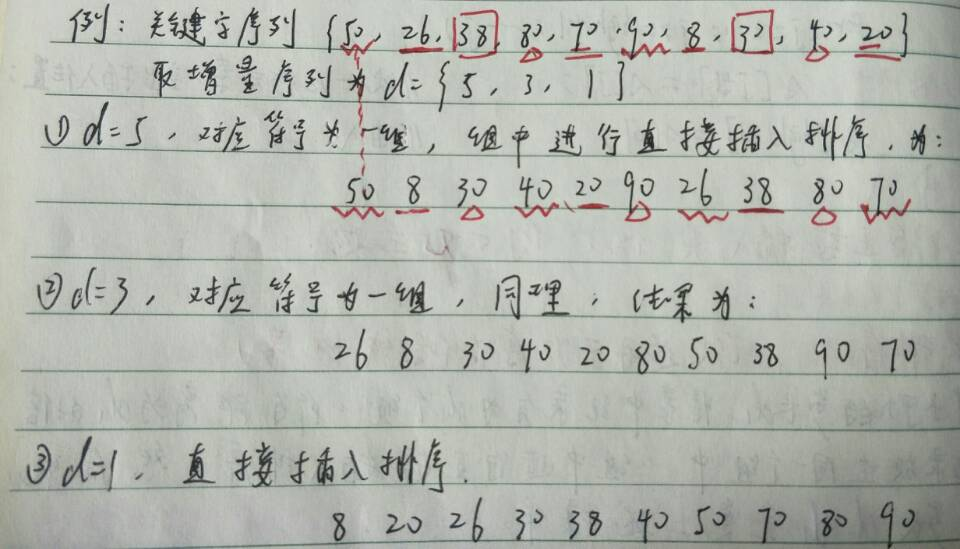## 冒泡排序（Bubble Sort）

### 算法流程 （递增为例）

1. 比较相邻的元素。如果第一个比第二个大，就交换他们两个
2. 对每一对相邻元素作同样的工作，从开始第一对到结尾的最后一对。在这一点，最后的元素应该会是最大的数
3. 针对所有的元素重复以上的步骤，除了最后一个
4. 持续每次对越来越少的元素重复上面的步骤，直到没有任何一对数字需要比较。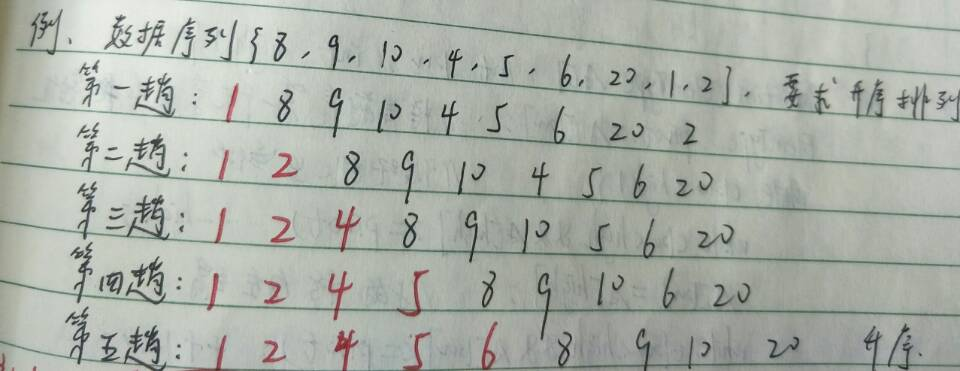### 拓展 （冒泡排序的改进 —- 鸡尾酒排序）

1. 先对数组从左到右进行升序的冒泡排序；
2. 再对数组进行从右到左的降序的冒泡排序；
3. 以此类推，持续的、依次的改变冒泡的方向，并不断缩小没有排序的数组范围；

## 快速排序（Quick Sort）

### 基本思想

1. 先从数列中取出一个数作轴值（基准数）pivot
2. 根据基准数将数列进行分区，小于基准数的放左边，大于基准数的放右边；
3. 重复分区操作，知道各区间只有一个数为止。

### 算法流程（递归+挖坑填数）

1. $i=L，j=R$，将基准数挖出形成第一个坑$a[i]$；
2. $j–$由后向前找出比它小的数，找到后挖出此数$a[j]$填到前一个坑$a[i]$中；
3. $i++$从前向后找出比它大的数，找到后也挖出此数填到前一个坑$a[j]$中；
4. 再重复2,3，直到$i=j$，将基准数填到$a[i]​$。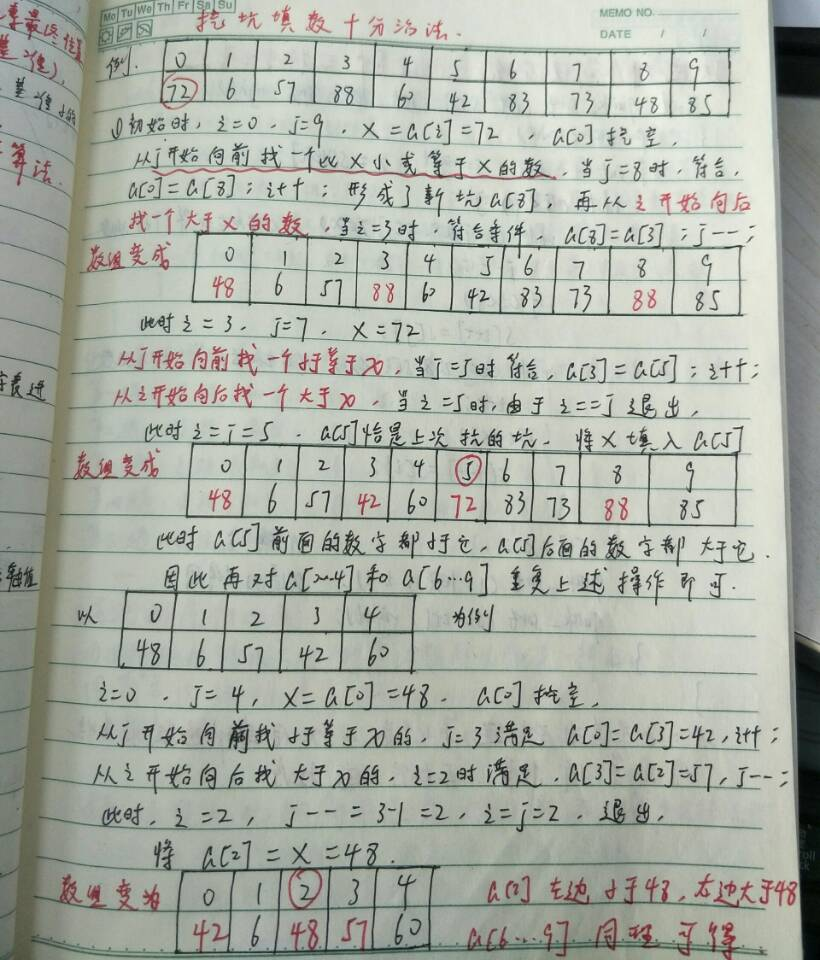### 算法实现

------ 本文结束------